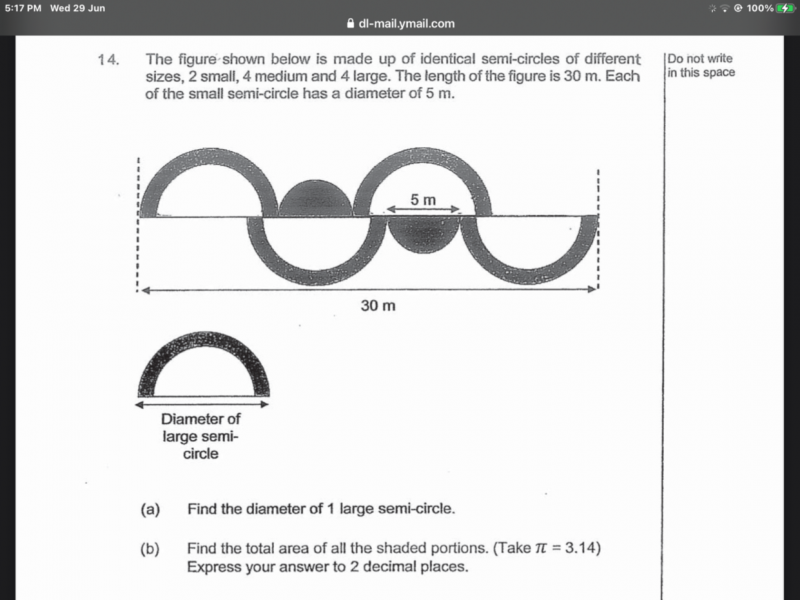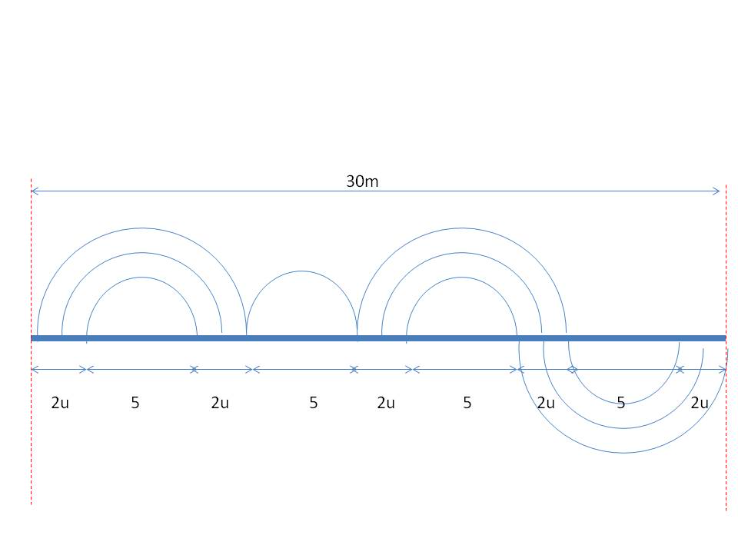# QuestionPls help to solve, thanksLet u be the width of the path created by the large and medium circles.

D of large circle = 2u + 5 + 2u

From the diagram,   5(2u) + 4(5) = 30 => 10u = 10 => u =1

(a)  Diameter of large circle = 4(1) + 5 = 9m

=> Radius of large circle = 4.5

(b)  Radius of medium circle =  [5 + 2(1)] / 2 = 3.5

Radius of small circle = 5/2 = 2.5

Area of shaded area = Area of 2 large circles – Area of 2 medium circles + Area of small circle

= 3.14 [ 2(4.5)2 – 2(3.5)2 + (2.5)2]

= 3.14 (40.5 – 24.5 + 6.25)

= 69.87 m(2 dec. places)